Courses
Courses for Kids
Free study material
Offline Centres
More

# NCERT Exemplar for Class 7 Science Solutions Chapter 13 Motion and TimeLast updated date: 02nd Dec 2023
Total views: 425.4k
Views today: 6.25k

## Class 7 Science NCERT Exemplar Solutions Chapter 13 Motion and Time

Free PDF download of NCERT Exemplar for Class 7 Science Chapter - 13 Motion and Time solved by expert Science teachers on Vedantu.com as per NCERT (CBSE) Book guidelines. All Chapter - 13 Motion and Time exercise questions with solutions to help you to revise the complete syllabus and score more marks in your examinations.

Vedantu offers NCERT Exemplar Science solutions for Class 7 and it benefits students who want to top their exams. They can study the different topics of a subject from any place they want by going through the chapter-wise solutions and PDFs offered by Vedantu. Students, as well as parents, can access these solutions from anywhere, just by being connected to the internet. They can go beyond to strengthen their study experience and practice the activities exclusively presented by Vedantu at their own will.

## Access NCERT Exemplar Solutions for Class 7 Science Chapter 13- MOTION AND TIME

### 1. Which of the following cannot be used for measurement of time? (a) A leaking tap. (b) Simple pendulum. (c) Shadow of an object during the day. (d) Blinking of eyesAns: (d) Blinking of eyesExplanation: Blinking eyes aren't something that happens all the time. As a result, it can't be used to measure time.2. Two clocks A and B are shown in Figure 13.1. Clock A has an hour and a minute hand, whereas clock B has an hour hand, minute hand as well as a second hand. Which of the following statement is correct for these clocks?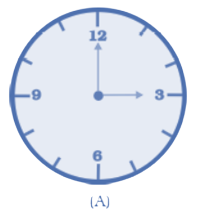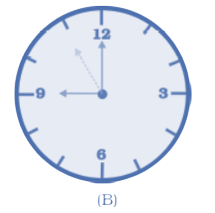(a) A time interval of 30 seconds can be measured by clock A.

(b) A time interval of 30 seconds cannot be measured by clock B.

(c) Time interval of 5 minutes can be measured by both A and B.

(d) Time interval of 4 minutes 10 seconds can be measured by clock A.

Ans: (c) Time intervals of 5 minutes can be measured by both A and B.

3. Two students were asked to plot a distance-time graph for the motion described by Table A and Table B.

 TABLE A Distance moved (m) 0 10 20 30 40 50 Time (minutes) 0 2 4 6 8 10

 TABLE B Distance moved (m) 0 5 10 15 20 25 Time (minutes) 0 1 2 3 4 5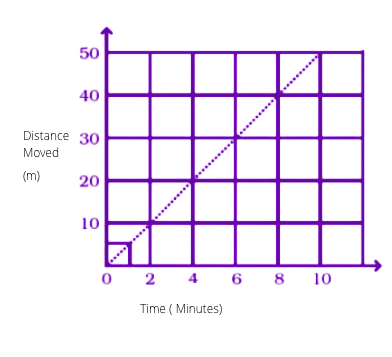The graph given in Figure 13.2 is true for

(a) Both A and B.

(b) A only.

(c) B only.

(d) Neither A nor B.

Ans: (a) Both A and B.

Explanation: The speed of A and B is constant according to the table.

4. A bus travels 54 km in 90 minutes. The speed of the bus is

(a) 0.6 m/s

(b) 10 m/s

(c) 5.4 m/s

(d) 3.6 m/s

Ans:  (b) 10 m/s

Explanation:

$\;Given,Distance\; = 54\;km = 54 \times 1000 = 54000\;{\text{m}}.\;Time\; = 90\;minutes\; = 90 \times 60 = 5400\;\sec .\;Speed\; = \frac{{\;Distance\;}}{{\;Time\;}} = \frac{{54000}}{{5400}} = 10\;{\text{m}}/{\text{s}}$

5. If we denote speed by S, distance by D and time by T, the relationship between these quantities is

(a) ${\text{S}}\; = \;{\text{D}}\; \times \;{\text{T}}$

(b)  ${\text{T}} = \frac{{\text{S}}}{{\text{D}}}$

(c) ${\text{S}}\; = \;\frac{1}{{\text{T}}}\; \times \;{\text{D}}$

(d) ${\text{S}}\; = \;\frac{{\text{T}}}{{\text{D}}}$

Ans: (c) ${\text{S}}\; = \;\frac{1}{{\text{T}}}\; \times \;{\text{D}}$

6. Observe Figure 13.3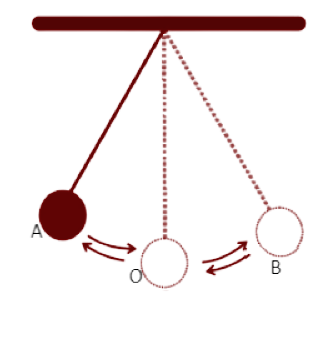The time period of a simple pendulum is the time taken by it to travel from

(a) A to B and back to A.

(b) O to A, A to B and B to A.

(c) B to A, A to B and B to O.

(d) A to B.

Ans: (a) A to B and back to A.

Explanation: The 'Time-period' is the amount of time it takes for the pendulum to complete a single oscillation.

7. Fig. 13.4 shows an oscillating pendulum.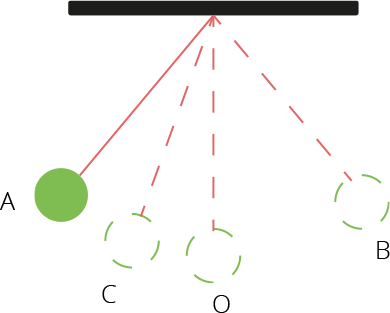Time taken by the bob to move from A to C is t1 and from C to O is t2. The time period of this simple pendulum is

(a) (t1 + t2

(b) 2 (t1 + t2

(c) 3 (t1 + t2 )

(d) 4 (t1 + t2

Ans: (d) 4 (t1 + t2

Explanation: The 'Time-period' is the amount of time it takes for the pendulum to complete a single oscillation. As a result, there is only one oscillation: A to C to O to B to O to C to A.

Time take A to O and O to B = Equal

So, the time period of simple pendulum is

$= \left( {{{\text{t}}_1} + {{\text{t}}_2}} \right) + \left( {{{\text{t}}_1} + {{\text{t}}_2}} \right) + \left( {{{\text{t}}_1} + {{\text{t}}_2}} \right) + \left( {{{\text{t}}_1} + {{\text{t}}_2}} \right) = 4\left( {{{\text{t}}_1} + {{\text{t}}_2}} \right)$

8. The correct symbol to represent the speed of an object is

(a) 5 m/s

(b) 5 mp

(c) 5 m/s-1

(d) 5 s/m

Ans: (a) 5 m/s

Explanation: Time is measured in seconds (s) and distance is measured in metres (m).

9. Boojho walks to his school which is at a distance of 3 km from his home in 30 minutes. On reaching he finds that the school is closed and comes back by a bicycle with his friend and reaches home in 20 minutes. His average speed in km/h is

(a) 8.3

(b) 7.2

(c) 5

(d) 3.6

Ans: (b) 7.2

Explanation:

$Average\;speed\; = \frac{{\;Total\;distance\;}}{{\;Total\;time\;taken\;}} = \frac{6}{{\frac{{50}}{{60}}}} = \frac{{6 \times 60}}{{50}} = 7.2\;km/{\text{h}}$

### VERY SHORT ANSWER TYPE QUESTIONS

10. A simple pendulum is oscillating between two points A and B as shown in Figure 13.5. Is the motion of the bob uniform or non-uniform?Ans:  Bob's motion is non-uniform because it does not cover the same distance in the same amount of time.

11. Paheli and Boojho have to cover different distances to reach their school but they take the same time to reach the school. What can you say about their speed?

Ans: Paheli and Boojho have different speeds. They traverse unequal distances in the same amount of time. When compared to another, the one who covers the greatest distance has the greatest speed.

12. If Boojho covers a certain distance in one hour and Paheli covers the same distance in two hours, who travels in a higher speed?

Ans: Travelling speed of Boojho is higher than Paheli.

13. Complete the data of the table given below with the help of the distance-time graph given in Figure 13.6.

 Distance (m) 0 4 ? 12 ? 20 Time (s) 0 2 4 ? 8 10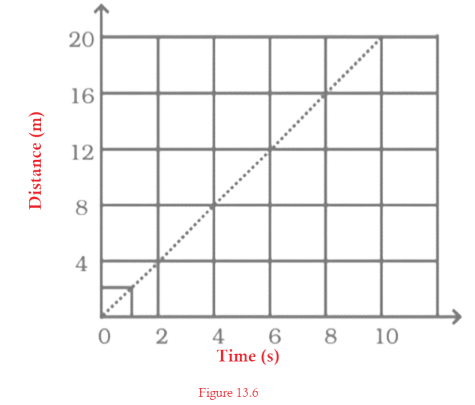Ans: According to the given graph

 Distance (m) 0 4 8 12 16 20 Time (s) 0 2 4 6 8 10

14. The average age of children of Class VII is 12 years and 3 months. Express this age in seconds.

Ans: $The\;average\;age\; = \;12\;years\;3\;months$

$1\;year\; = \;365\;Days\;$

$= \;365\; \times \;24\;hours\;\left[ {\;\because \;1\;days\; = \;24\;hours} \right]$

$= \;365\; \times \;24\; \times \;3600\;\sec \;\left[ {\;\because \;1\;hour\; = \;3600\;\sec } \right]\; = \;31536000\;\sec$

$So,\;12\;years\; = \;31536000\;\sec \; \times \;12\; = \;378432000\;seconds\;$

$And\;3\;months\; = \;3\; \times \;30\;days\; = \;3\; \times \;30\; \times \;24\;hours\; = \;3\; \times \;30\; \times \;24\; \times \;3600{\text{s}}\; = \;7776000\;seconds$

$Now,\;the\;total\;age\;in\;seconds\; = \;378432000{\text{s}}\; + \;7776000\;{\text{s}}\; = \;386208000{\text{s}}$

15.  A spaceship travels 36,000 km in one hour. Express its speed in km/s.

Ans: $Given,Distance\; = \;36000\;km,\;and\;\;Time\; = \;1\;hour\;\;\left[ {\;\because \;1\;hour\; = \;3600\;\sec } \right]\;As\;per\;formula,\;Speed\; = \frac{{\;Distance}}{{\;Time\;}} = \frac{{36000}}{{1 \times 3600}} = 10\;km/{\text{s}}$

16. Starting from A, Paheli moves along a rectangular path ABCD as shown in Figure 13.7. She takes 2 minutes to travel each side. Plot a distance-time graph and explain whether the motion is uniform or non-uniform.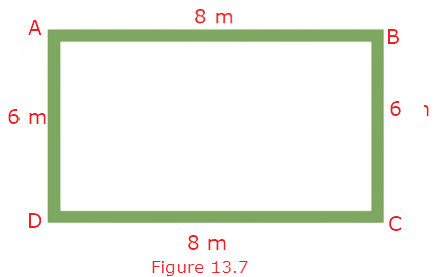Ans: Because the distance travelled per unit time for the full distance traversed is not equal, the motion is non-uniform. The graph is as follows: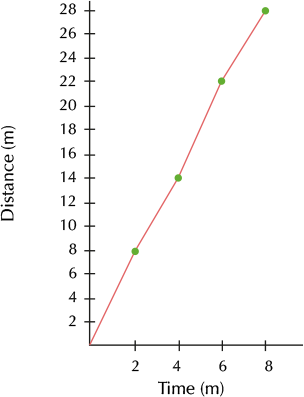17. Plot a distance-time graph of the tip of the second hand of a clock by selecting 4 points on x-axis and y-axis respectively. The circumference of the circle traced by the second hand is 64 cm.

Ans: According to the specified conditions, an equivalent distance is covered in an equal amount of time. As a result, the graph will consist of a straight line.

 Time (s) x 15 30 45 60 Distance (cm) y 16 32 48 64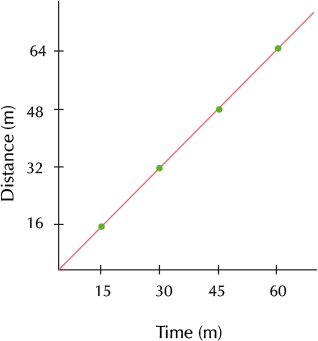### 18.  Given below as Figure 13.8 is the distance-time graph of the motion an object.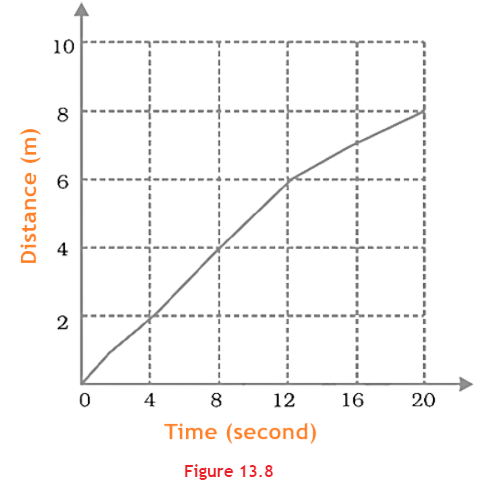i) What will be the position of the object at 20s?

Ans: The position of the object at 20s is 8m.

ii) What will be the distance travelled by the object in 12s?

Ans: The distance travelled by the object in 12s is 6m.

iii) What is the average speed of the object?

Ans: The average speed of an object is:

$\;Average\;Speed\; = \frac{{\;Total\;Distance\;}}{{\;Total\;time\;}} = \frac{8}{{20}} = 0.4\;{\text{m}}/{\text{s}}$

19. Distance between Bholu’s and Golu’s house is 9 km. Bholu has to attend Golu’s birthday party at 7 o’clock. He started from his home at 6 o’clock on his bicycle and covered a distance of 6 km in 40 minutes. At that point he met Chintu and he spoke to him for 5 minutes and reached Golu’s birthday party at 7 o’clock. With what speed did he cover the second part of the journey? Calculate his average speed for the entire journey.

Ans: As per the question, speed of Bholu:

$\;Speed\; = \frac{{\;Distance\;}}{{\;Time\;}} = \frac{{3\;km}}{{\frac{{15}}{{60}}\;{\text{h}}}} = \frac{3}{{15}} \times 60 = 12\;km/{\text{h}}$

Now,

$\;Average\;speed\; = \frac{{\;Total\;distance\;travelled\;}}{{\;Total\;time\;taken\;}} = \frac{9}{1} = 9\;km/{\text{h}}$

20. Boojho goes to the football ground to play football. The distance time graph of his journey from his home to the ground is given as Figure 13.9.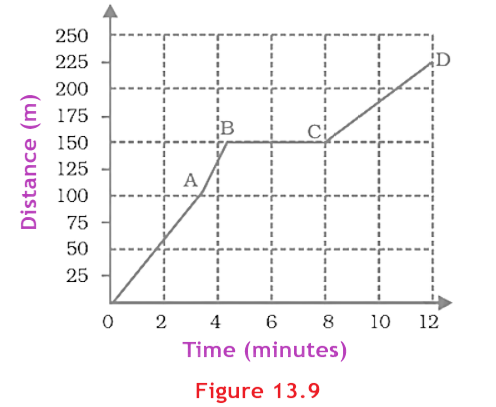(a) What does the graph between point B and C indicate about the motion of Boojho?

Ans: The graph indicates that there is no motion between point B and C. Boojho will be at rest.

(b) Is the motion between 0 to 4 minutes uniform or non uniform?

Ans: The motion between 0 to 4 minutes will be non-uniform.

(c) What is his speed between 8 and 12 minutes of his journey?

Ans: As per the question,

$\;Speed\; = \frac{{\;Distance\;}}{{\;Time\;}} = \frac{{225 - 150}}{{12 - 8}} = \frac{{75\;{\text{m}}}}{{4\;minutes\;}}$

### NCERT Chapter 13 - Time and Motion

NCERT Chapter 13 - Time and Motion of Class 7  is one of the major chapters of physics that deals with the principles of speed, including different types of speed and motion and their calculations. This chapter provides information on the utilizations of speed and motion in our day to day life. The NCERT textbooks include examples of real-life situations where the principles of time and motion are applied so that the students understand the topic with ease and interest.

NCERT Motion and Time Chapter cover all the important topics of this physics Chapter. It starts with Slow and Fast-moving objects. We can decide if an object is fast or slow by the distance moved by the objects in a certain span of time. Then we move on to the concept of ‘Speed’, which can be explained by a fixed distance covered by a certain object in a unit of time. The difference between uniform speed and non-uniform speed is also mentioned in this part. We also learn to solve problems of measurement of time and time period; and measurement of speed and learn to draw the distance-time graph. There are varied activities included in the NCERT textbook to explain further the concepts of difference in speed, oscillatory motion (the time period of a simple pendulum), speed and motion of different objects, etc. These experiments help the students understand the mathematical part of physics and solve it easily. The NCERT textbooks also offer activities and projects on making your own Sundial and models of Sun clocks which provides the student with a way to enjoy chapters as well.

## FAQs on NCERT Exemplar for Class 7 Science Solutions Chapter 13 Motion and Time

1. What are the important formulas to learn from NCERT Class 7 Science Chapter 13?

Some of the important formulas to learn from NCERT Chapter 13 - Time and Motion are :

• The formula to calculate speed is Speed = Distance/ Time. We can decide the speed of a given thing once we can scale the time held by it to cover a given distance.

• Once you have the speed of an object, you can detect the distance moved by it in a given time. Then you have to multiply the speed by time. Distance covered = Speed × Time.

•  Likewise one can also detect the time an object would take to cover a distance while moving at a presented speed. Time taken = Distance/ Speed.

2. Why is studying Motion and Time so important?

All our activities depend on time. For instance, to see the duration of travel, to meet the timetables at work, to know whether it's daytime or nighttime, to know the heartbeat, to know the measure of time taken by the computer to accomplish an operation etc. Hence it's really important to measure the time. As students study this unit, they're going to find out how these physical factors impact their lives and work. Students will likewise learn to explain the location, understand perspective, and use simple machines to assist make their work easier.

3. Why should students consider Vedantu NCERT Solutions for Class 7 Science Chapter 13 Time and Motion?

Vedantu offers NCERT solutions both from the exercise and inside the chapter extra questions. As you can download the PDF formats from Vedantu it becomes easier to follow the exercises by downloading the content and going through it even when you lack internet access. At Vedantu only the most skilled teachers and educators frame the questions and solutions helping you to reach your potential. Vedantu offers you the best free education notes and solutions to all your NCERT questions.

4. How does Vedantu help you to understand the numerical from Chapter-13 of NCERT Solutions for Class 7 Science easily?

You can get good marks from this chapter if you clearly understand the mathematical part. To understand the methods of solving sums effortlessly, students are recommended to refer to the NCERT Solutions from Vedantu. The problems are solved in a systematic manner with explanations provided for every and step. Students can check with these solutions and solve the textbook problems with no difficulty. Short cut tips and tricks are highlighted within the solutions to assist students to remember the formulas during revision.

5. Why are following NCERT textbooks so important?

NCERT books are designed by a panel of teachers at NCERT who have tremendous teaching experience, knowledge of the syllabus, knowledge of the most effective teaching standards for students. They design NCERT books and study material that is in line with the latest syllabus. They make sure that the content within the books is written and explained in such a way that it can be read and understood by students of the whole country with various background intellectual levels.